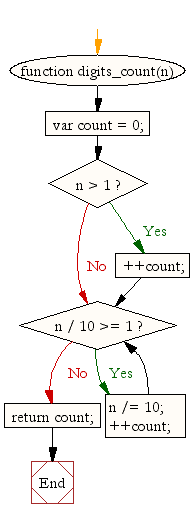# JavaScript: Count the digits of an integer

## JavaScript Math: Exercise-41 with Solution

Write a JavaScript function to count integer digits.

Sample Solution:-

HTML Code:

``````<!DOCTYPE html>
<html>
<meta charset="utf-8">
<title>JavaScript function to count the digits of an integer.</title>
<body>

</body>
</html>
```
```

JavaScript Code:

``````function digits_count(n) {
var count = 0;
if (n >= 1) ++count;

while (n / 10 >= 1) {
n /= 10;
++count;
}

return count;
}

console.log(digits_count(12112));

console.log(digits_count(457));
```
```

Sample Output:

```5
3
```

Flowchart:Live Demo:

See the Pen javascript-math-exercise-41 by w3resource (@w3resource) on CodePen.